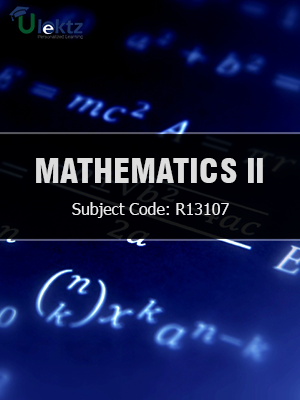•My WalletMy Order
•My Profile
•My Connections
•My Books
•My Videos
•My Tests
•My Calender
•My Messages
•My Shopping Cart
•My Orders
•Account Settings
•Help

# Book Details# Mathematics - II

 Course Code : R13107 Author : uLektz University : JNTU Kakinada Regulation : 2016 Categories : Electronics & Communication Format :ePUB3 (DRM Protected) Type : eBook

FREE

Description :Mathematics - II of R13107 covers the latest syllabus prescribed by JNTU Kakinada for regulation 2016. Author: uLektz, Published by uLektz Learning Solutions Private Limited.

Note : No printed book. Only ebook. Access eBook using uLektz apps for Android, iOS and Windows Desktop PC.

##### Topics
###### UNIT I Solution of Algebraic and Transcendental Equations

1.1 Introduction- Bisection Method

1.2 Method of False Position

1.3 Iteration Method

1.4 Newton-Raphson Method(One variable and Simultaneous Equations)

###### UNIT II Interpolation

2.1 Introduction- Errors in Polynomial Interpolation

2.2 Finite differences

2.3 Forward Differences- Backward differences -Central differences

2.4 Symbolic relations and separation of symbols-Differences of a polynomial

2.5 Newton’s formulae for interpolation

2.6 Interpolation with unevenly spaced points -Lagrange’s Interpolation formula

###### UNIT III Numerical solution of Ordinary Differential equations

3.1 Solution by Taylor’s series

3.2 Picard’s Method of successive Approximations

3.3 Euler’s Method

3.4 Runge-Kutta Methods

###### UNIT IV Fourier Series

4.1 Introduction- Determination of Fourier coefficients

4.2 Even and odd functions –change of interval

4.3 Half-range sine and cosine series application: Amplitude, spectrum of a periodic function

###### UNIT V Fourier Transforms:

5.1 Fourier integral theorem (only statement)

5.2 Fourier sine and cosine integrals - sine and cosine transforms –properties – inverse transform- Finite Fourier transforms

###### UNIT VI Z-transform:

6.1 Introduction– properties – Damping rule – Shifting rule - Initial and final value theorems

6.2 Inverse z transform

6.3 Convolution theorem

6.4 Solution of difference equation by Z -transforms.